图像处理连通分量_图像处理连通分量是什么 - CSDN
• %提取连通分量测试图像，用来检测食物中的外来物 I=im2double(imread('D:\Gray Files\9-18a.tif')); %获得图像大小 [M,N]=size(I); %存放腐蚀后的图像 %设定提取门限，根据经验设定 th=0.808; %将原图像根据上述门限...
clc;
clear all;
close all;
%提取连通分量测试图像，用来检测食物中的外来物
%获得图像大小
[M,N]=size(I);
%存放腐蚀后的图像
%设定提取门限，根据经验设定
th=0.808;
%将原图像根据上述门限进行二值处理，大于等于门限为1，小于为0
ind=find(I>=th);
I(ind)=1;
ind=find(I<th);
I(ind)=0;
%获得值为1的x,y坐标
[rows,cols]=find(I==1);
%将x,y坐标点组合成下标矩阵
index=cat(2,rows,cols);
%5*5结构元素
n=5;
B=ones(n,n);
n_l=floor(n/2);
%对边界图进行扩充，四周各加2行、2列0（与结构元素的大小相对应），目的是为了处理边界点
%将下标矩阵加2
index=index+2;
%保存所有连通对象集
Objs={};
%设定递归上限
set(0,'RecursionLimit',10000);
%递归寻找连通对象
while 1
%取出下标矩阵中的第一个值
if isempty(index)
break;
end
%取出下标矩阵中的第一个对象
p=index(1,:);
%将边界线对象保存至对象集
Objs=cat(1,Objs,A{1,1}-n_l);
index=A{1,2};
end
%显示连通对象
J=zeros(M,N);
if ~isempty(Objs)
for i=1:size(Objs,1)
Obj=Objs{i,1};
for j=1:size(Obj,1)
J(Obj(j,1),Obj(j,2))=1;
end
end
end
imshow(J)

FindConnectedPoint函数如下：

function R=FindConnectedPoint(p,Beta_pad,ind,n_l)
%保存边界坐标对象
Obj=[];
%以p为中心点，从边界图中取出3*3大小的块区域
Obj=p;
%将图像中的p点置零
%将块区域中的p点置零
Block(n_l+1,n_l+1)=0;
A=cell(1,3);
%将p点从下标矩阵中删除
for j=1:size(ind,1)
if ind(j,:)==p
ind(j,:)=[];
break;
end
end
%寻找块区域中为1的点的下标
[rows,cols]=find(Block==1);
ind_sub=cat(2,rows,cols);
if ~isempty(ind_sub)
%确定块中数值为1的点的坐标
for i=1:size(ind_sub,1)
p_next=[];
if ind_sub(i,1)<=n_l+1
p_next(1,1)=p(1,1)-abs(n_l+1-ind_sub(i,1));
if ind_sub(i,2)<=n_l+1
p_next(1,2)=p(1,2)-abs(n_l+1-ind_sub(i,2));
else
p_next(1,2)=p(1,2)+abs(n_l+1-ind_sub(i,2));
end
else
p_next(1,1)=p(1,1)+abs(n_l+1-ind_sub(i,1));
if ind_sub(i,2)<=n_l+1
p_next(1,2)=p(1,2)-abs(n_l+1-ind_sub(i,2));
else
p_next(1,2)=p(1,2)+abs(n_l+1-ind_sub(i,2));
end
end
Obj=cat(1,A{1,1},Obj);
end
%更新下标矩阵
ind=A{1,2};
end
end
%返回结果
end


展开全文灰度图像处理
• %% 第9章 形态学处理 %% imdilate膨胀 clc clear A1=imread('.\images\dipum_images_ch09\Fig0906(a)(broken-text).tif'); info=imfinfo('.\images\dipum_images_ch09\Fig0906(a)(broken-text).tif') B=[0 1 0 1 ...
<span style="font-size:18px;">%% 第9章 形态学处理

%% imdilate膨胀
clc
clear

info=imfinfo('.\images\dipum_images_ch09\Fig0906(a)(broken-text).tif')
B=[0 1 0
1 1 1
0 1 0];
A2=imdilate(A1,B);%图像A1被结构元素B膨胀
A3=imdilate(A2,B);
A4=imdilate(A3,B);

subplot(221),imshow(A1);
title('imdilate膨胀原始图像');

subplot(222),imshow(A2);
title('使用B后1次膨胀后的图像');

subplot(223),imshow(A3);
title('使用B后2次膨胀后的图像');

subplot(224),imshow(A4);
title('使用B后3次膨胀后的图像');
27%imdilate图像膨胀处理过程运行结果如下：

%% imerode腐蚀
clc
clear
subplot(221),imshow(A1);
title('腐蚀原始图像');

%strel函数的功能是运用各种形状和大小构造结构元素
se1=strel('disk',5);%这里是创建一个半径为5的平坦型圆盘结构元素
A2=imerode(A1,se1);
subplot(222),imshow(A2);
title('使用结构原始disk(5)腐蚀后的图像');

se2=strel('disk',10);
A3=imerode(A1,se2);
subplot(223),imshow(A3);
title('使用结构原始disk(10)腐蚀后的图像');

se3=strel('disk',20);
A4=imerode(A1,se3);
subplot(224),imshow(A4);
title('使用结构原始disk(20)腐蚀后的图像');
%图像腐蚀处理过程运行结果如下：

%% 开运算和闭运算
clc
clear
%se=strel('square',5');%方型结构元素
se=strel('disk',5');%圆盘型结构元素
imshow(f);%原图像
title('开闭运算原始图像')
61%运行结果如下：

%开运算数学上是先腐蚀后膨胀的结果
%开运算的物理结果为完全删除了不能包含结构元素的对象区域，平滑
%了对象的轮廓，断开了狭窄的连接，去掉了细小的突出部分
fo=imopen(f,se);%直接开运算
figure,subplot(221),imshow(fo);
title('直接开运算');

%闭运算在数学上是先膨胀再腐蚀的结果
%闭运算的物理结果也是会平滑对象的轮廓，但是与开运算不同的是，闭运算
%一般会将狭窄的缺口连接起来形成细长的弯口，并填充比结构元素小的洞
fc=imclose(f,se);%直接闭运算
subplot(222),imshow(fc);
title('直接闭运算');

foc=imclose(fo,se);%先开后闭运算
subplot(223),imshow(foc);
title('先开后闭运算');

fco=imopen(fc,se);%先闭后开运算
subplot(224),imshow(fco);
title('先闭后开运算');
84%开闭运算结果如下：

%先膨胀再腐蚀
fse=imdilate(f,se);%膨胀

%gcf为得到当前图像的句柄，当前图像是指例如PLOT,TITLE,SURF等
%get函数为得到物体的属性，get(0,'screensize')为返回所有物体screensize属性值
%set函数为设置物体的属性
figure,set(gcf,'outerposition',get(0,'screensize'));%具体目的是设置当前窗口的大小
subplot(211),imshow(fse);
title('使用disk(5)先膨胀后的图像');

fes=imerode(fse,se);
subplot(212),imshow(fes);
title('使用disk(5)先膨胀再腐蚀后的图像');
99%先膨胀后腐蚀图像如下：

%先腐蚀再膨胀
fse=imerode(f,se);
figure,set(gcf,'outerposition',get(0,'screensize'))
subplot(211),imshow(fse);
title('使用disk(5)先腐蚀后的图像');

fes=imdilate(fse,se);
subplot(212),imshow(fes);
title('使用disk(5)先腐蚀再膨胀后的图像');
110%先腐蚀后膨胀的图像如下：

%% imopen imclose在指纹上的应用
clc
clear
se=strel('square',3);%边长为3的方形结构元素
subplot(121),imshow(f);
title('指纹原始图像');

A=imerode(f,se);%腐蚀
subplot(122),imshow(A);
title('腐蚀后的指纹原始图像');
123%指纹原始图像和腐蚀后的图像结果如下：

fo=imopen(f,se);
figure,subplot(221),imshow(fo);
title('使用square(3)开操作后的图像');

fc=imclose(f,se);
subplot(222),imshow(fc);
title('使用square闭操作后的图像');

foc=imclose(fo,se);
subplot(223),imshow(foc);
title('使用square(3)先开后闭操作后的图像')

fco=imopen(fc,se);
subplot(224),imshow(fco);
title('使用square(3)先闭后开操作后的图像');
140%指纹图像开闭操作过程结果如下：

%% bwhitmiss击中或击不中变换
clc
clear
imshow(f);
title('击中或不击中原始图像');
148%击中或不击中原始图像显示结果如下：

B1=strel([0 0 0;0 1 1;0 1 0]);%击中：要求击中所有1的位置
B2=strel([1 1 1;1 0 0;1 0 0]);%击不中，要求击不中所有1的位置
B3=strel([0 1 0;1 1 1;0 1 0]);%击中
B4=strel([1 0 1;0 0 0;0 0 0]);%击不中
B5=strel([0 0 0;0 1 0;0 0 0]);%击中
B6=strel([1 1 1;1 0 0;1 0 0]);%击不中

g=imerode(f,B1)&imerode(~f,B2)%利用定义来实现击中或击不中
figure,subplot(221),imshow(g);
title('定义实现组1击中击不中图像');

g1=bwhitmiss(f,B1,B2);
subplot(222),imshow(g1);
title('结构数组1击中击不中后的图像');

g2=bwhitmiss(f,B3,B4);
subplot(223),imshow(g2);
title('结构数组2击中击不中的图像');

g3=bwhitmiss(f,B5,B6);
subplot(224),imshow(g3);
title('结构数组3击中击不中的图像');
172%击中击不中变换后图像如下：

%%makelut
clc
clear

f=inline('sum(x(:))>=3');%inline是用来定义局部函数的
lut2=makelut(f,2)%为函数f构造一个接收2*2矩阵的查找表
lut3=makelut(f,3)

%% Conway生命游戏
clc
clear
lut=makelut(@conwaylaws,3);
bw1=  [0     0     0     0     0     0     0     0     0     0
0     0     0     0     0     0     0     0     0     0
0     0     0     1     0     0     1     0     0     0
0     0     0     1     1     1     1     0     0     0
0     0     1     0     0     0     0     1     0     0
0     0     1     0     1     1     0     1     0     0
0     0     1     0     0     0     0     1     0     0
0     0     0     1     1     1     1     0     0     0
0     0     0     0     0     0     0     0     0     0
0     0     0     0     0     0     0     0     0     0  ];
subplot(221),imshow(bw1,'InitialMagnification','fit');
title('Generation 1');

bw2=applylut(bw1,lut);
subplot(222),imshow(bw2,'InitialMagnification','fit'),
title('Generation 2');

bw3=applylut(bw2,lut);
subplot(223),imshow(bw3,'InitialMagnification','fit');
title('Generation 3');

temp=bw1;
for i=2:100
bw100=applylut(temp,lut);
temp=bw100;
end
subplot(224),imshow(bw100,'InitialMagnification','fit')
title('Generation 100');
214%显示Generation结果如下：

%% getsequence
clc
clear
se=strel('diamond',5)
decomp=getsequence(se)%getsequence函数为得到分解的strel序列
decomp(1)
decomp(2)

%% endpoints
clc
clear

subplot(121),imshow(f1);
title('原始形态骨架图像');

g1=endpoints(f1);
%set(gcf,'outerposition',get(0,'screensize'));%运行完后自动生成最大的窗口
subplot(122),imshow(g1);
title('骨架图像的端点图像');
%骨架头像端点检测头像如下：

figure,subplot(121),imshow(f2);
title('原始骨头图像');

g2=endpoints(f2);
subplot(122),imshow(g2);
title('骨头图像端点头像');%结果是没有端点
245%骨头头像端点检测图像如下：

%% bwmorph组合常见形态学之细化
clc
clear
subplot(221),imshow(f);
title('指纹图像细化原图');

g1=bwmorph(f,'thin',1);
subplot(222),imshow(g1);
title('指纹图像细化原图');

g2=bwmorph(f,'thin',2);
subplot(223),imshow(g2);
title('指纹图像细化原图');

g3=bwmorph(f,'thin',Inf);
subplot(224),imshow(g3);
title('指纹图像细化原图');
265%指纹图像细化过程显示如下：

%% bwmorph组合常见形态学之骨骼化
clc
clear
subplot(131),imshow(f);
title('指纹图像骨骼化原图');

fs=bwmorph(f,'skel',Inf);
subplot(132),imshow(fs);
title('指纹图像骨骼化');

for k=1:5
fs=fs&~endpoints(fs);
end
subplot(133),imshow(fs);
title('指纹图像修剪后骨骼话');
283%指纹图像骨骼化过程显示：

%% 使用函数bwlabel标注连通分量
clc
clear
imshow(f),title('标注连通分量原始图像');
290%其结果显示如下：

[L,n]=bwlabel(f);%L为标记矩阵，n为找到连接分量的总数
[r,c]=find(L==3);%返回第3个对象所有像素的行索引和列索引

rbar=mean(r);
cbar=mean(c);

figure,imshow(f)
hold on%保持当前图像使其不被刷新
for k=1:n
[r,c]=find(L==k);
rbar=mean(r);
cbar=mean(c);
plot(cbar,rbar,'Marker','o','MarkerEdgeColor','k',...
'MarkerFaceColor','k','MarkerSize',10);%这个plot函数用法不是很熟悉
plot(cbar,rbar,'Marker','*','MarkerFaceColor','w');%其中的marker为标记
end
title('标记所有对象质心后的图像');

%% 由重构做开运算
clc
clear
subplot(321),imshow(f);
title('重构原始图像');

fe=imerode(f,ones(51,1));%竖线腐蚀
subplot(322),imshow(fe);
title('使用竖线腐蚀后的结果');

fo=imopen(f,ones(51,1));%竖线做开运算
subplot(323),imshow(fo);
title('使用竖线做开运算结果');

fobr=imreconstruct(fe,f);%fe做标记
subplot(324),imshow(fobr);
title('使用竖线做重构开运算');

ff=imfill(f,'holes');%对f进行孔洞填充
subplot(325),imshow(ff);
title('对f填充孔洞后的图像');

fc=imclearborder(f,8);%清除边界，2维8邻接
subplot(326),imshow(fc);
title('对f清除边界后的图像');
336%图像重构过程显示如下：

%% 使用顶帽变换和底帽变换
clc
clear
subplot(221),imshow(f);
title('顶帽底帽变换原始图像');

se=strel('disk',10);%产生结构元素
%顶帽变换是指原始图像减去其开运算的图像
%而开运算可用于补偿不均匀的背景亮度，所以用一个大的结构元素做开运算后
%然后用原图像减去这个开运算，就得到了背景均衡的图像，这也叫做是图像的顶帽运算
f1=imtophat(f,se);%使用顶帽变换
subplot(222),imshow(f1);
title('使用顶帽变换后的图像');

%底帽变换是原始图像减去其闭运算后的图像
f2=imbothat(imcomplement(f),se);%使用底帽变换，为什么原图像要求补呢？
%f2=imbothat(f,se);%使用底帽变换
subplot(223),imshow(f2);
title('使用底帽变换后的图像');

%顶帽变换和底帽变换联合起来用，用于增加对比度
subplot(224),imshow(f3);
title('使用顶帽底帽联合变换后图像');
363%顶帽底帽变换过程图像如下：

%%使用开运算和闭运算做形态学平滑
%由于开运算可以除去比结构元素更小的明亮细节，闭运算可以除去比结构元素更小的暗色细节
%所以它们经常组合起来一起进行平滑图像并去除噪声
clc
clear
subplot(221),imshow(f);
title('木钉图像原图');

se=strel('disk',5);%disk其实就是一个八边形
fo=imopen(f,se);%经过开运算
subplot(222),imshow(f);
title('使用半径5的disk开运算后的图像');

foc=imclose(fo,se);
subplot(223),imshow(foc);
title('先开后闭的图像');

fasf=f;
for i=2:5
se=strel('disk',i);
fasf=imclose(imopen(fasf,se),se);
end
subplot(224),imshow(fasf);
title('使用开闭交替滤波后图像');
390%使用开运算和闭运算做形态学平滑结果如下：

%% 颗粒分析
clc
clear

sumpixels=zeros(1,36);
for k=0:35
se=strel('disk',k);
fo=imopen(f,se);
sumpixels(k+1)=sum(fo(:));
end

%可以看到，连续开运算之间的表面积会减少
plot(0:35,sumpixels),xlabel('k'),ylabel('surface area');
title('表面积和结构元素半径之间的关系');
407%其运算结果如下：

figure,plot(-diff(sumpixels));%diff()函数为差分或者近似倒数，即相邻2个之间的差值
xlabel('k'),ylabel('surface area reduction');
title('减少的表面积和结构元素半径之间的关系');
412%其运算结果如下：

%% 使用重构删除复杂图像的背景
clc
clear
subplot(221),imshow(f);
title('灰度级重构原图像');

f_obr=imreconstruct(imerode(f,ones(1,71)),f);
subplot(222),imshow(f_obr);
title('经开运算重构图');

f_o=imopen(f,ones(1,71));
subplot(223),imshow(f_o);
title('经开运算后图');

f_thr=imsubtract(f,f_obr);
subplot(224),imshow(f_thr);
title('顶帽运算重构图')
432%使用重构删除复杂图像的背景1:

f_th=imsubtract(f,f_o)
figure,subplot(221),imshow(f_th);
title('经顶帽运算图');

g_obr=imreconstruct(imerode(f_thr,ones(1,11)),f_thr);
subplot(222),imshow(g_obr);
title('用水平线对f_thr经开运算后重构图');

g_obrd=imdilate(g_obr,ones(1,2));
subplot(223),imshow(g_obrd);
title('使用水平线对上图进行膨胀');

f2=imreconstruct(min(g_obrd,f_thr),f_thr);
subplot(224),imshow(f2);
title('最后的重构结果');
449%使用重构删除复杂图像的背景2:</span>
展开全文• 提取连通分量的过程实际上也是标注连通分量的过程，通常的做法是给原图像中的每个连通区分配一个唯一代表该区域的编号，在输出图像中该连通区内的所有的像素值就赋值为该区域的编号，我们将这样的输出图像称为标注...
基本概念
提取连通分量的过程实际上也是标注连通分量的过程，通常的做法是给原图像中的每个连通区分配一个唯一代表该区域的编号，在输出图像中该连通区内的所有的像素值就赋值为该区域的编号，我们将这样的输出图像称为标注图像。这里介绍一种基于形态学的膨胀操作的提取连通分量的方法。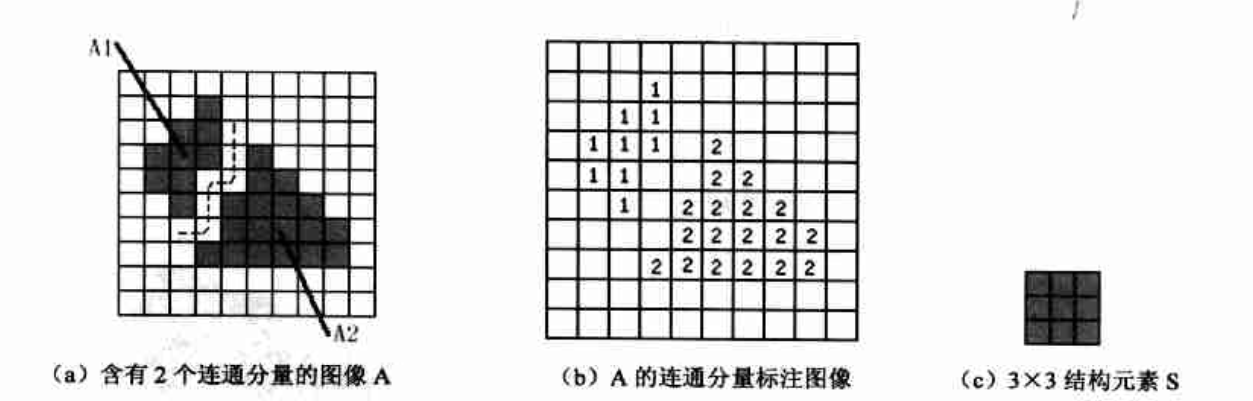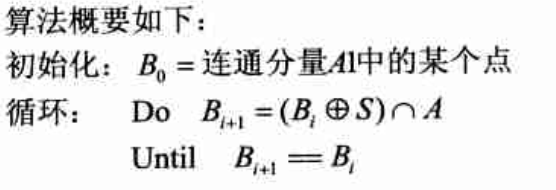示例演示
我们用OpenCV实现连通分量提取函数，然后进行细菌计数。完整工程代码。
/*
*only process binary image
*iseight = true means eight connected, otherwise four connected
*/
int LabelConnectedComponent(const cv::Mat &src, cv::Mat &dst, bool iseight = true)
{
Mat structelement;
if(iseight)
structelement = getStructuringElement(MORPH_RECT, Size(3, 3));
else
structelement = getStructuringElement(MORPH_CROSS, Size(3, 3));

dst = Mat::ones(src.size(), src.type());
Mat tmp = Mat::ones(src.size(), src.type()); // save last reuslt image
Mat img = Mat::ones(src.size(), src.type()); //image B
int labelnum = 0; //label of connected component

Mat backupsrc;
src.copyTo(backupsrc);
for(int i = 0; i < backupsrc.rows; i++)
{
for(int j = 0; j < backupsrc.cols; j++)
{
if(backupsrc.at<uchar>(i, j) == 255)
{
Mat img = Mat::ones(src.size(), src.type());
img.at<uchar>(i, j) = 255;
img.copyTo(tmp);  //Temporary save
labelnum++;

while(true)  // until not change
{
cv::dilate(img, img, structelement);
bitwise_and(img, src, img);
//if img do not change, this connected component is finished
if (cv::countNonZero(img - tmp) == 0)
break;
img.copyTo(tmp);
}

//label the connected component
for(int r = 0; r < img.rows; r++)
{
for(int c = 0; c < img.cols; c++)
{
if(img.at<uchar>(r, c) == 255)
{
backupsrc.at<uchar>(r, c) = 0;
dst.at<uchar>(r, c) = labelnum;
}
}
}
}
}
}
return labelnum;
}

先对原始图像进行膨胀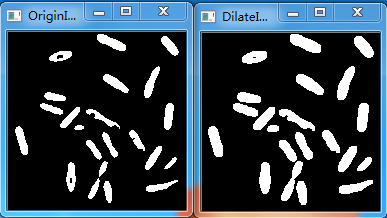然后进行细菌统计，实际的细菌个数为21。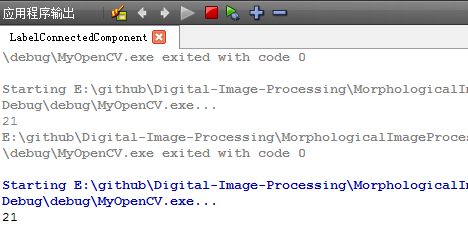展开全文连通分量 连通区域 连通分量标记
• 连通成分是给二值图像中的每个连通区域标上一个特定标号的算法，该算法可以用来对图像中的目标进行定位和计数。 邻域： 中间像素与他周围像素之间的位置关系决定了是几邻域连接，如下图表示了4邻域与8邻域 算法思路...
连通成分的标记原理
连通成分是给二值图像中的每个连通区域标上一个特定标号的算法，该算法可以用来对图像中的目标进行定位和计数。
邻域：
中间像素与他周围像素之间的位置关系决定了是几邻域连接，如下图表示了4邻域与8邻域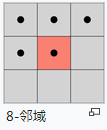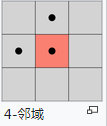算法思路
使用的数据结构为队列，用元组模仿队列，将像素的坐标值入队
1、从左到右，从上到下遍历每一个像素，判断该像素的像素值是否为1，并且判断它是否被标记过，如果没有被标记过并且像素值为1，则将该像素的位置入队。
2、查找该像素的8邻域内有没有像素值为1的并且没有被标记过的像素，有的话则给与它与该像素一样的标记，并且将该像素入队。
3、每次判断完一个像素的8邻域之后要记得将队列头加一。
4、队列为空之后即表示一个连通区域标记完成。则换一种标记，将队列置空，接着进行其他像素的判断。
Matlab代码
s=[0 0 0 0 0 0 0 0 0 0 0 0 0 0 0 0 0;
0 0 1 1 0 0 1 1 0 0 1 1 0 0 1 1 0;       %这个矩阵是维基百科中的矩阵
0 1 1 1 1 1 1 1 1 0 0 1 1 1 1 0 0;
0 0 0 1 1 1 1 0 0 0 1 1 1 1 0 0 0;
0 0 1 1 1 1 0 0 0 1 1 1 0 0 1 1 0;
0 1 1 1 0 0 0 0 0 0 0 1 1 1 0 0 0;
0 0 1 1 0 0 0 0 0 1 0 0 0 0 0 1 0;
0 0 0 0 0 0 1 1 1 1 0 0 1 1 1 1 0;
0 0 0 0 0 0 0 0 0 0 0 0 0 0 0 0 0];

imshow(mat2gray(s));
[m n]=size(s);
tmp=zeros(m,n);     %标记图像
label=1;
queue_tail=1;       %队列尾
neighbour=[-1 -1;-1 0;-1 1;0 -1;0 1;1 -1;1 0;1 1];  %和当前像素坐标相加得到八个邻域坐标

for i=2:m-1
for j=2:n-1

if s(i,j)==1 && tmp(i,j) ==0
tmp(i,j)=label;
q{queue_tail}=[i j];        %用元组模拟队列，当前坐标入列
queue_tail=queue_tail+1;

for k=1:8               %8邻域搜索
pix1=pix+neighbour(k,:);
if pix1(1)>=2 && pix1(1)<=m-1 && pix1(2) >=2 &&pix1(2)<=n-1  %边界条件的限定
if s(pix1(1),pix1(2)) == 1 && tmp(pix1(1),pix1(2)) ==0  %如果当前像素邻域像素为1并且标记图像的这个邻域像素没有被标记，那么标记
tmp(pix1(1),pix1(2))=label;
q{queue_tail}=[pix1(1) pix1(2)];
queue_tail=queue_tail+1;
end
end
end
end

clear q;                %清空队列，为新的标记做准备
label=label+1;
queue_tail=1;
end

end
end
figure,imshow(mat2gray(tmp))

实验结果比较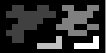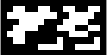展开全文• ## 连通分量的提取

千次阅读 2017-02-28 22:34:54
应用背景：在许多自动图像分析应用中，如何识别出图像中的不同之处，往往是从其二值图像中提取连通分量连通分量的定义：令S是一个像素子集，如果S中的全部像素之间存在一个通路（m通路或8通路），则可以说两个...图像处理 matlab 连通分量
• 图像的保存形式 一幅图像，经过取样和量化之后就可以得到一幅数字图像。数字图像在在保存时，都是由单一的像素保存在...数字图像处理的基本操作，有些需要在空间域中进行，而另外的一些则需要在变换域中进行。 空间域：
• ## 连通分量标记算法

千次阅读 2016-05-15 18:55:09
图像分割领域中一个重要步骤是求取图像连通区域，后续图像轮廓理解的基石。连通区域标记
• VC数字图像处理编程讲座之十 图像几何特性分析一、物体边界计算和记数　（一）邻接和连通　邻接和连通是图像的基本几何特性之一图像处理 算法 扩展 c
• 击中：击中其实是一种模板匹配，如果一个结构元（模板）与图像中的一个连通区域完全相等，那么腐蚀的结果将是一个点，这就算是“击中”了，因为找到了完全一致的模式（模式的含义去查一下吧，这里的模式和模式识别的...
• regionprops统计被标记的区域的面积分布，显示区域总数。 配合[L,num]=bwlabel(bw,8); %另一篇博文里有函数regionprops语法规则为： STATS = regionprops(C,properties) ...EquivDiameter
• java图像处理之图的联通计数 图的联通分量计数，根据连通区域可分为八连通和四连通 （1）八连通：上左，上，上右，左，右，下左，下，下右 1 1 1 1 0 1 1 1 1 0和周围的1都视为连通关系 （2）四连通：上，下，...java 图像处理 递归算法
• 所谓的连通分量， 就是无向图中的极大连通子图。 对于连通图， 只有一个连通分量， 就是它本身。 非联通的无向图有多个连通分量。   如下图， 该无向图的连通分量分别是： (A L M J B F C)，
• 形态学图像处理（一）预备知识1.1 预备知识1.1.1 集合理论中的基本概念1.2 二值图像、集合及逻辑算子（二）膨胀和腐蚀2.1 膨胀2.2 结构元的分解2.3 strel函数2.4 腐蚀（三） 膨胀与腐蚀的结合3.1 开操作和闭操作3.2 ...形态学图像处理 膨胀
• 《数字图像处理》的第二章，主要讲了图像的一些定义，数字图像处理的基本概念和方法。本章节为后面做具体处理提供了最基本的概念。读书笔记 图像处理
• 第九章 形态学图像处理 第九章 形态学图像处理 一腐蚀和膨胀 1 腐蚀 2 膨胀 二开操作与闭操作 三击中或击不中变换 四一些基本的形态学算法 1 边界提取 2 孔洞填充 3 连通分量的提取 4 凸壳 5 细化 6 粗化 7 ...数字图像处理 腐蚀和膨胀 开操作和闭操作
• 计算二值图像连通区域的中心点/质心， 演示Image Moments算法与连通组件标记算法运用 二值图像区域中心点寻找在图像分析与模式识别中有着重要的地位。图像处理 算法 JAVA Image
...